# Corporate Financial Accounting Study Set 3

## Quiz 9 : Long Term Assets: Fixed and IntangibleStudy FlashcardsLooking for Accounting Homework Help?

## Quiz 9 :Long Term Assets: Fixed and Intangible

Question TypeComparing three depreciation methods Dexter Industries purchased packaging equipment on January 8 for $72,000, The equipment was expected to have a useful life of three years, or 18,000 operating hours, and a residual value of$4,500, The equipment was used for 7,600 hours during Year 1, 6,000 hours in Year 2, and 4,400 hours in Year 3. Instructions 1. Determine the amount of depreciation expense for the three years ending December 31, by (A) the straight-line method, (B) the units-of-activity method, and (C) the double-declining-balance method. Also determine the total depreciation expense for the three years by each method. The following columnar heading are suggested for recording the depreciation expense amounts: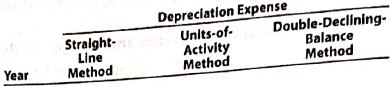2. What method yields the highest depreciation expense for Year 1? 3. What method yields the most depreciation over the three-year life of the equipment?
Free
Essay

Depreciation
It is the expense that creates charge on the cost of the asset over its estimated useful life. It is the value that indicates the reduction in the assets' usage ability with the passage of time. There are various methods to determine this value over different kinds of assets used by the business units.
1
In the present case, Equipment is purchased by D industry at $72,000; estimated useful life is 3 years or 18,000 hours with$4,500 as its residual value.
Calculation of depreciation expense for 3 years is given as under.
A
Straight-line method: This method provides the value of depreciation which remains constant over the useful life of the asset. The value of depreciation will be computed on the cost of the asset every year.
So, the depreciation value for the current year is calculated as below.The depreciation amount by the straight-line method remains constant over its useful life unless the revision of its elements has done.
Hence, it is concluded that the annual depreciation for each of the year is $22,500 B Unit of activity Method: This method indicates the value of depreciation charged over actual units involved in the activities on standard basis. Calculation of depreciation for the equipment can be done by this method would followed by two steps as given below. Firstly the depreciation per unit is calculated as under.Now, the expense for the depreciation is computed as under. For the first yearTherefore, depreciation for the first year by this method is$28,500.
For the second yearTherefore, depreciation for the second year by this method is $22,500. For the third yearTherefore, depreciation for the third year by this method is$16,500.
C
Double-declining method: This method helps in the valuation of depreciation which declines over the useful life of the asset. This method is generally used where the consumption rate of the asset is rapid. It can be calculated by using the formula given under.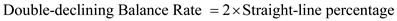Firstly, the straight- line percentage is computed as under.By putting the value of SLP in above formula of double-declining balance rate, it would be 66.6% i.e.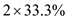.
Therefore, the annual depreciation for the first year by this method would be computed by formula given under.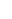Therefore, depreciation for the first year by this method is $47,952.Now, the book value of equipment on Jan 1, would be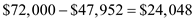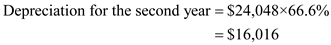Therefore, depreciation for the second year by this method is$16,016.Now, the book value of equipment on Jan 1, would be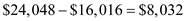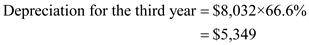Therefore, depreciation for the third year by this method is $5,349. The table as below mentioned shown the calculation of depreciation as per different methods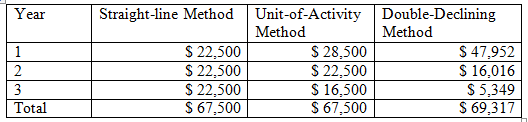2 For first year, the highest expense of depreciation has been charged with amount of$47,952. As straight-line method stands $22,500 and unit-of-activity methods stands at$28,500, both shows the lower amount than double- declining method.
Therefore, it is concluded that double-declining method yields highest value of depreciation for the first year.
3
Over the useful life of the equipment, the double-declining method yields the highest value of depreciation with the amount of $69,317. While the straight-line method and unit-of-activity methods stand at total of$67,500 as depreciation expense, which is lower amount than double- declining method.Therefore, it is concluded that double-declining method yields highest value of depreciation over three years of life of equipment.

Tags
Choose question tagUnits-of-activity depreciation A truck acquired at a cost of $69,000 has an estimated residual value of$12,000, has an estimated useful life of 300,000 miles, and was driven 77,000 miles during the year. Determine (A) the depreciable cost, (B) the depreciation rate, and (C) the units-of-activity depreciation for the year.
Free
Essay

Unit of activity method
This method is one of the methods that are used to calculate the depreciation expense for the asset. It evaluates the depreciation amount in the terms of activities-unit involved during usage of the asset by the business. If the tractor is taken as asset for an example, the activity-unit will be usage hours to compute depreciation.
In the present case, the cost of truck is $69,000; estimated residual value is$12,000. Being the miles in this case is taken as unit of activity for the truck; the estimated useful life is 300,000 miles. And the operating miles covered during the year is 77,000 miles.
A
Depreciable cost: It is the cost that represents the difference between the cost of the asset and its salvage value. The resultant amount will show the cost for which the depreciation expenses need to calculate.
So, the depreciable cost would be determined by using the formula as given below.Hence, it is concluded that the depreciable cost is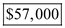B
Depreciation rate: The rate of depreciation which measures the operating speed of activity in terms of units defined for the asset. It is usually denoted in per unit value.
So, the rate by this method can be computed by putting the values in the formula as given under.Hence it can be concluded that the depreciation rate is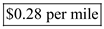C
Depreciation by the Unit of activity: It indicates the value of depreciation charged over actual units involved in the activities on standard basis.
Calculation of depreciation for the truck can be done by this method would followed by two steps as given below.
Firstly the depreciation per unit is calculated as under.Now, the expense for the depreciation is computed as under.Hence, it is concluded that the depreciation for the year is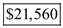.

Tags
Choose question tagStraight-line depreciation A building acquried at the beginning of the year at a cost of $1,450,000 has an estimated residual value of$300,000 and an estimated useful life of 10 years. Determine (A) the depreciable cost, (B) the straight-line rate, and (C) the annual straight-line depreciation.
Free
Essay

Straight-line depreciation
This depreciation is the value which assumes the constant wear-tear loss of assets over its useful life. It is the form of expense that is charged on the cost of asset by the business units. It provides the simplest way to match expenses with revenue earned from the asset used in the normal course of the business.
In the present case, the book-value of the building is $1,450,000; estimated useful life is 10 years with$300,000 as its residual value.
A
Depreciable cost: It is the cost that represents the difference between the cost of the asset and its salvage value. The resultant amount will show the cost for which the depreciation expenses that need to be calculate.
So, the depreciable cost would be determined by using the formula as given below.Hence, it is concluded that the depreciable cost is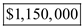B
Straight-line rate: It shows the rate of depreciation which remains same for each of the accounting period. It represents the assumed speed of usage of an asset during the normal operations of the business unit. It is usually denoted in percentage value.
So, the rate by this method can be computed by putting the values in the formula as given under.Hence, it is concluded that the rate of straight-line method is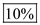C
Annual straight-line depreciation: This depreciation is the value which assumes the annual reduction in the value of assets equally throughout its useful life.
It can be determined by dividing the depreciable cost to estimated useful life of the asset which is shown as below.Hence, it is concluded that the annual depreciation is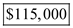Tags
Choose question tagDepreciation by three methods; partial years Layton Company purchased tool sharpening equipment on October 1 for $108,000. The equipment was expected to have a useful life of three years or 12,000 operating hours, and a residual value of$7,200. The equipment was used for 1,350 hours during Year 1, 4,200 hours in Year 2, 3,650 hours in Year 3, and 2,800 hours in Year 4. Instructions Determine the amount of depreciation expense for the years ended December 31, Year 1, Year 2, Year 3, and Year 4, by (A) the straight-line method, (B) the units-of-activity method, and (C) the double-declining-balance method.
Essay
Tags
Choose question tagTeam Activity In teams, select a public company that interests you. Obtain the company's most recent annual report on Form 10-K. The Form 10-K is a company's annually required filing with the Securities and Exchange Commission (SEC). It includes the company's financial statements and accompanying notes. The Form 10-K can be obtained either (A) from the Investor relations section of the company's Web site or (B) by using the company search feature of the SEC's EDGAR database service found at www.sec.gov/edgar/searchedgar/companyscarch.html. 1. Based on the information in the company's most recent annual report, answer the following questions: A. What deprecition methods does the company use to compute deprecition expense? B. How much deprecition expense does the company report on its income statements? C. What is the intial cost of the company's fixed assets? D. What is the book value of the company's fixed assets? E. What types of intangible assets, if any, does the company report on its balance sheet? 2. Does the book value of the company' fixed assets reflect their current market value? Explain your answer.
Essay
Tags
Choose question tagFedEx and UPS: Fixed asset turnover ratio FedEx Corporation and United Parcel Service, Inc. compete in the package delivery business. The major fixed assets for each business include aircraft, sorting and handling facilities, delivery vehicles, and information technology. The sales and average book value of fixed assets reported on recent financial statements for each company were as follows: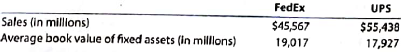A. Compute the fixed asset turnover ratio for each company. (Round to one decimal place.) B. Which company appears more efficient in using fixed assets? C. Interpret the meaning of the ratio for the more efficient company.
Essay
Tags
Choose question tagContinuing Company Analysis-Amazon: Fixed asset turnover ratio Amazon.com, Inc. is the world's leading Internet retailer of merchandise and media. Amazon also designs and sells electronic products, such as e-readers. Netflix, Inc. is the world's leading Internet television network. Both companies compete in the digital media and streaming space. However, Netfix is more narrowly focused in the digital streaming business than is Amozon. Sales and average book value of fixed assets information (in millions) are provided Amozon and Netflix for a recent year as follows: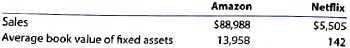A. Compute the fixed asset turnover ratio for each company. (Round to one decimal place.) B. Which company is more efficient in generating sales from fixed assets? C. Interpret your results.
Essay
Tags
Choose question tagDepreciation by three methods; partial Years Perdue Company purchased equipment on April 1 for $270,000. The equipment was expected to have a useful life of three years or 18,000 operating hours, and a residual value of$9,000. The equipment was used for 7,500 hours during Year 1, 5,500 hours in Year 2, 4,000 hours in Year 3, and 1,000 hours in Year 4. Instructions Determine the amount of depreciation expense for the years ended December 31, Year 1, Year 2, Year 3, and Year 4, by (A) the straight-line method, (B) the units-of-activity method, and (C) the double-declining-balance method.
Essay
Tags
Choose question tagBullwinkle Co. acquired an adjacent vacant lot with the hope of selling it in the future at a gain. The lot is not intended to be used in Bullwinkle business operations. Where should such real estate be listed on the balance sheet?
Essay
Tags
Choose question tagAllocating payments and receipts to fixed asset accounts The following payments and receipts are related to land, land improvements, and buildings acquired for use in a wholesale ceramic business. The receipts are identified by an asterisk.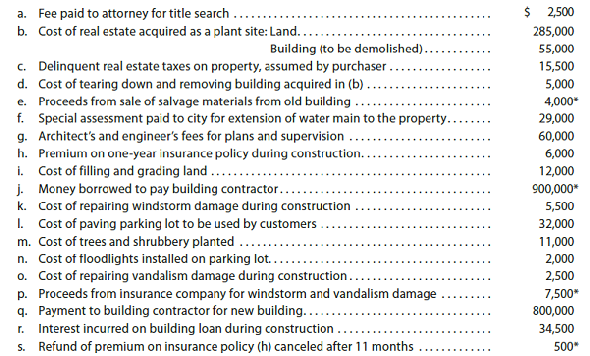Instructions 1. Assign each payment and receipt to Land (unlimited life), Land Improvements (limited life), Building, or Other Accounts. Indicate receipts by an asterisk. Identify each item by letter and list the amounts in columnar form, as follows: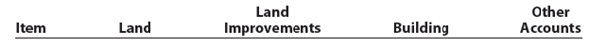2. Determine the amount debited to Land, Land Improvements, and Building. 3. The costs assigned to the land, which is used as a plant site, will not be depreciated, while the costs assigned to land improvements will be depreciated. Explain this seemingly contradictory application of the concept of depreciation. 4. What would be the effect on the current year's income statement and balance sheet if the cost of filling and grading land of $12,000 [payment (i)] was incorrectly classified as Land Improvements rather than Land? Assume that Land Improvements are depreciated over a 20-year life using the double-declining-balance method. Essay Answer: Tags Choose question tagDouble-declining-balance depreciation A building acquired at the beginning of the year at a cost of$1,375,000 has an estimated residual value of $250,000 and an estimated useful life of 40 years. Determine (A) the double-declining-balance rate and (B) the double-declining-balance depreciation for the first year. Essay Answer: Tags Choose question tagComparing three depreciation methods Waylander Coatings Company purchased waterproofing equipment on January 6 for$320,000. The equipment was expected to have a useful life of four years, or 20,000 operating hour, an a residual value of $35,000. The equipment was used for 7,200 hours during Year 1, 6,400 hours in Year 2, 4,400 hours in Year 3, and 2,000 hours in Year 4. Instructions 1. Determine the amount of depreciation expense for the years ended December 31, Year 1, Year 2, Year 3, and Year 4, by (A) the straight-line method, (B) the units-of-activity method, and (C) the double-declining-balance method. Also determine the total depreciation expense for the four years by each method. The following columnar headings are suggested for recording the depreciation expense amounts: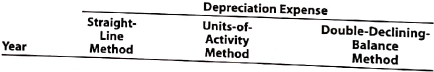2. What method yield the highest depreciation expense for Year 1? 3. What method yields the most depreciation over the four-year life of the equipment? Essay Answer: Tags Choose question tagEthics in Action Hard Bodies Co. is a fitness chain that has just completed its second year of operations. At the beginnig of its first fiscal year, the company purchased fitness equipment at a cost of$600,000 and estimated that the equipment would have a useful life of five years and no residual value. The company uses the straight-line depreciation method. The company reported net income for the first two years of operations as follows: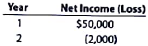Mike Gambit, the company's chief financial officer (CFO), has recently run financial models to predict future net income, and he expects net losses to continue at $(2,000) per year for the next three years. James Steed, the president of Hard Bodies, is concerned about these predictions, as he is under pressure from the company's owner to return the company to Year 1 net income levels. If the company does not meet these goals, both he and Mike will likely be fired. Mike suggests that the company change the estimated useful life of the fitness equipment to 10 years and increase the equipment's estimated residual value to$50,000. This will reduce depreciation expense and increase net income. 1. Evaluate the decision to change the equipment's estimated useful life and estimated residual value to improve earnings. How does this change impact the usefulness of the company's net income for external decision makers? 2. If Mike and James make the change, are they acting in an ethical manner? Explain.
Essay
Tags
Choose question tagKeyser Company purchased a machine that has a manufacturer's suggested life of 20 years. The company plans to use the machine on a special project that will last 12 years. At the completion of the project, the machine will be sold. Over how many years should the machine be depreciated?
Essay
Tags
Choose question tagVerizon: Fixed asset turnover ratio Verizon Communications Inc. is a major telecommunications company in the United States. Two recent balance sheet for Verizon disclosed the following information regarding fixed assets: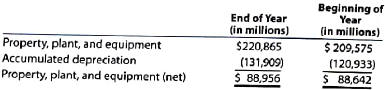Verizon's revenue for the year was $120,550 million. Assume the fixed asset turnover ratio for the telecommunications industry averages approximately 1.1. A. Determine Verizon's fixed asset turnover ratio. (Round to one decimal place.) B. Interpret this ratio with respect to the industry average. Essay Answer: Tags Choose question tagDetermining cost of land Bridger Ski Co. has developed a tract of land into a ski resort. The company has cut the trees, cleared and graded the land and hills, and constructed ski lifts. (a) Should the tree cutting, land clearing, and grading costs of constructing the ski slopes be debited to the land account? (b) If such costs are debited to Land, should they be depreciated? Explain. Essay Answer: Tags Choose question tagDetermining cost of land On-Time Delivery Company acquired an adjacent lot to construct a new warehouse, paying$90,000 and giving a short-term note for $50,000. Legal fees paid were$1,750 delinquent taxes assumed were $25,000, and fees paid to remove an old building from the land were$9,000. Materials salvaged from the demolition of the building were sold for $1,000. A contractor was paid$415,000 to construct a new warehouse. Determine the cost of the land to be reported on the balance sheet.
EssayAllocating payments and receipts to fixed asset accounts The following payments and receipts are related to land, land improvements, and buildings acquired for use in a wholesale apparel business. The receipts are identified by an asterisk.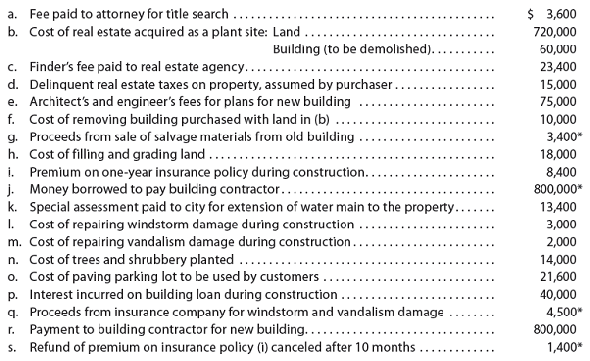Instructions 1. Assign each payment and receipt to Land (unlimited life), Land Improvements (limited life), Building, or Other Accounts. Indicate receipts by an asterisk. Identify each item by letter and list the amounts in columnar form, as follows: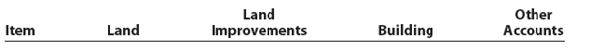2. Determine the amount debited to Land, Land Improvements, and Building. 3. The costs assigned to the land, which is used as a plant site, will not be depreciated, while the costs assigned to land improvements will be depreciated. Explain this seemingly contradictory application of the concept of depreciation. 4. What would be the effect on the current year's income statement and balance sheet if the cost of paving the parking lot of $21,600 [payment (o)] was incorrectly classified as Land rather than Land Improvements? Assume that Land Improvements are depreciated over a 10-year life using the double-declining-balance method. Essay Answer: Tags Choose question tagCosts of acquiring fixed assets Melinda Stoffers owns and operates ABC Print Co. During February, ABC incurred the following costs in acquiring two printing presses. One printing press was new, and the other was purchased from a business that recently filed for bankruptcy. Costs related to new printing press: 1. Fee paid to factory representative for installation 2. Freight 3. Insurance while in transit 4. New parts to replace those damaged in unloading 5. Sales tax on purchase price 6. Special foundation Costs related to used printing press: 7. Fees paid to attorney to review purchase agreement 8. Freight 9. Installation 10. Repair of damage incurred in reconditioning the press 11. Replacement of worn-out parts 12. Vandalism repairs during installation a. Indicate which costs incurred in acquiring the new printing press should be debited to the asset account. b. Indicate which costs incurred in acquiring the used printing press should be debited to the asset account. Essay Answer: Tags Choose question tagAlpine Company solicited bids from several contractors to construct an addition to its office building. The lowest bid received was for$1,200,000. Alpine decided to construct the addition itself at a cost of \$1,100,000. What amount should be recorded in the building account?